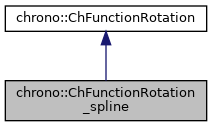chrono::ChFunctionRotation_spline Class Reference

## Description

A rotation function q=f(s) that interpolates n rotations using a "quaternion spline" of generic order.

For order 1 (linear) this boils down to a classical SLERP interpolation. For higher orders, this operates as in the paper "A C^2-continuous B-spline quaternion curve interpolating a given sequence of solid orientations", Myoung-Jun Kim, Myung-Soo Kim. 1995. DOI:10.1109/CA.1995.393545. Note, except for order 1 (linear) the rotation does not pass through control points, just like for positions in Bspline, except for first and last point. Exact interpolation requires the 'inversion' of the control points (to do).

#include <ChFunctionRotation_spline.h>

Inheritance diagram for chrono::ChFunctionRotation_spline:[legend]
Collaboration diagram for chrono::ChFunctionRotation_spline:[legend]

## Public Member Functions

ChFunctionRotation_spline ()
Constructor. By default constructs a linear SLERP between two identical null rotations.

ChFunctionRotation_spline (int morder, const std::vector< ChQuaternion<> > &mrotations, ChVectorDynamic<> *mknots=0)
Constructor from a given array of control points; each control point is a rotation to interpolate. More...

ChFunctionRotation_spline (const ChFunctionRotation_spline &other)

virtual ChFunctionRotation_splineClone () const override
"Virtual" copy constructor.

double ComputeUfromKnotU (const double u) const
When using Evaluate() etc. More...

double ComputeKnotUfromU (const double U) const
When using Evaluate() etc. More...

std::vector< ChQuaternion<> > & Rotations ()
Access the rotations, ie. quaternion spline control points.

ChVectorDynamicKnots ()
Access the knots.

int GetOrder ()
Get the order of spline.

virtual void SetupData (int morder, const std::vector< ChQuaternion<> > &mrotations, ChVectorDynamic<> *mknots=0)
Initial easy setup from a given array of rotations (quaternion spline control points). More...

std::shared_ptr< ChFunctionGetSpaceFunction () const
Gets the address of the function u=u(s) telling how the curvilinear parameter u of the spline changes in s (time).

void SetSpaceFunction (std::shared_ptr< ChFunction > m_funct)
Sets the function u=u(s) telling how the curvilinear parameter of the spline changes in s (time). More...

void SetClosed (bool mc)
Set as closed periodic spline: start and end rotations will match at 0 and 1 abscyssa as q(0)=q(1), and the Evaluate() and Derive() functions will operate in periodic way (abscyssa greater than 1 or smaller than 0 will wrap to 0..1 range). More...

bool GetClosed ()
Tell if the rotation spline is closed periodic.

virtual ChQuaternion Get_q (double s) const override
Return the q value of the function, at s, as q=f(s). More...

virtual void ArchiveOUT (ChArchiveOut &marchive) override
Return the derivative of the rotation function, at s, expressed as angular velocity w in local frame. More...

virtual void ArchiveIN (ChArchiveIn &marchive) override
Method to allow de-serialization of transient data from archives.Public Member Functions inherited from chrono::ChFunctionRotation
ChFunctionRotation (const ChFunctionRotation &other)

virtual ChVector Get_w_loc (double s) const
Return the derivative of the rotation function, at s, expressed as angular velocity w in local frame. More...

virtual ChVector Get_a_loc (double s) const
Return the derivative of the rotation function, at s, expressed as angular acceleration in local frame. More...

virtual void Estimate_s_domain (double &smin, double &smax) const
Return an estimate of the domain of the function argument. More...

virtual void Update (const double t)
Update could be implemented by children classes, ex. to launch callbacks.

## ◆ ChFunctionRotation_spline()

 chrono::ChFunctionRotation_spline::ChFunctionRotation_spline ( int morder, const std::vector< ChQuaternion<> > & mrotations, ChVectorDynamic<> * mknots = 0 )

Constructor from a given array of control points; each control point is a rotation to interpolate.

Input data is copied. If the knots are not provided, a uniformly spaced knot vector is made.

Parameters
 morder order p: 1= linear, 2=quadratic, etc. mrotations control points, size n. Each is a rotation. Required: at least n >= p+1 mknots knots, size k. Required k=n+p+1. If not provided, initialized to uniform.

## ◆ ArchiveOUT()

 void chrono::ChFunctionRotation_spline::ArchiveOUT ( ChArchiveOut & marchive )
overridevirtual

Return the derivative of the rotation function, at s, expressed as angular velocity w in local frame.

Return the derivative of the rotation function, at s, expressed as angular acceleration in local frame. Method to allow serialization of transient data to archives

Reimplemented from chrono::ChFunctionRotation.

## ◆ ComputeKnotUfromU()

 double chrono::ChFunctionRotation_spline::ComputeKnotUfromU ( const double U ) const
inline

When using Evaluate() etc.

you need U parameter to be in 0..1 range, but knot range is not necessarily in 0..1. So you can convert U->u, where u is in knot range, calling this:

## ◆ ComputeUfromKnotU()

 double chrono::ChFunctionRotation_spline::ComputeUfromKnotU ( const double u ) const
inline

When using Evaluate() etc.

you need U parameter to be in 0..1 range, but knot range is not necessarily in 0..1. So you can convert u->U, where u is in knot range, calling this:

## ◆ Get_q()

 ChQuaternion chrono::ChFunctionRotation_spline::Get_q ( double s ) const
overridevirtual

Return the q value of the function, at s, as q=f(s).

Parameter s always work in 0..1 range, even if knots are not in 0..1 range. So if you want to use s in knot range, use ComputeUfromKnotU().

Implements chrono::ChFunctionRotation.

## ◆ SetClosed()

 void chrono::ChFunctionRotation_spline::SetClosed ( bool mc )

Set as closed periodic spline: start and end rotations will match at 0 and 1 abscyssa as q(0)=q(1), and the Evaluate() and Derive() functions will operate in periodic way (abscyssa greater than 1 or smaller than 0 will wrap to 0..1 range).

The closure will change the knot vector (multiple start end knots will be lost) and will create auxiliary p control points at the end that will be wrapped to the beginning control points.

## ◆ SetSpaceFunction()

 void chrono::ChFunctionRotation_spline::SetSpaceFunction ( std::shared_ptr< ChFunction > m_funct )
inline

Sets the function u=u(s) telling how the curvilinear parameter of the spline changes in s (time).

Otherwise, by default, is a linear ramp, so evaluates the spline from begin at s=0 to end at s=1

## ◆ SetupData()

 void chrono::ChFunctionRotation_spline::SetupData ( int morder, const std::vector< ChQuaternion<> > & mrotations, ChVectorDynamic<> * mknots = 0 )
virtual

Initial easy setup from a given array of rotations (quaternion spline control points).

Input data is copied. If the knots are not provided, a uniformly spaced knot vector is made.

Parameters
 morder order p: 1= linear, 2=quadratic, etc. mrotations rotations, size n. Required: at least n >= p+1 mknots knots, size k. Required k=n+p+1. If not provided, initialized to uniform.

The documentation for this class was generated from the following files:
• /builds/uwsbel/chrono/src/chrono/motion_functions/ChFunctionRotation_spline.h
• /builds/uwsbel/chrono/src/chrono/motion_functions/ChFunctionRotation_spline.cpp